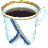# JScheme

Implementation of programming language Lisp

JScheme is Scheme interpreter developed by Ken Anderson, Tim Hickey and Peter Norvig. The language is almost compliant with R4RS. JScheme is written in Java and provides a very simple interface with it. It is distributed under zlib/libpng license.JScheme logo

## Examples:

### Hello, World!:

Example for versions JScheme 7.2, MIT/GNU Scheme 7.7.9, guile 1.8.5

Printing a message is a side effect of this command. Depending on the chosen implementation, the command will return either the message printed, or `Unspecified return value`.

``````(write "Hello, World!")
``````

### Factorial:

Example for versions JScheme 7.2, MIT/GNU Scheme 7.7.9, guile 1.8.5

This example uses tail-recursive factorial calculation. Note that GNU Guile and MIT/GNU Scheme print correct result, while JScheme has values of factorial starting with 13! wrong due to overflow.

``````(define (factorial x)
(if (< x 2)
1
(* x (factorial (- x 1)))))

(do ((i 0 (+ i 1)))
((> i 16))
(display (string-append (number->string i) "! = "))
(display (number->string (factorial i)))
(newline))
``````

### Fibonacci numbers:

Example for versions JScheme 7.2, MIT/GNU Scheme 7.7.9, guile 1.8.5

This example uses recursive definition of Fibonacci numbers.

``````(define (fibonacci x)
(if (< x 2)
x
(+ (fibonacci (- x 1)) (fibonacci (- x 2)))))

(do ((i 1 (+ i 1)))
((> i 16))
(display (string-append (number->string (fibonacci i)) ", ")))
(display "...")
(newline)
``````